# In the figure shown below ml-4 kg and m2- 2kg. Neglect the friction between ml and...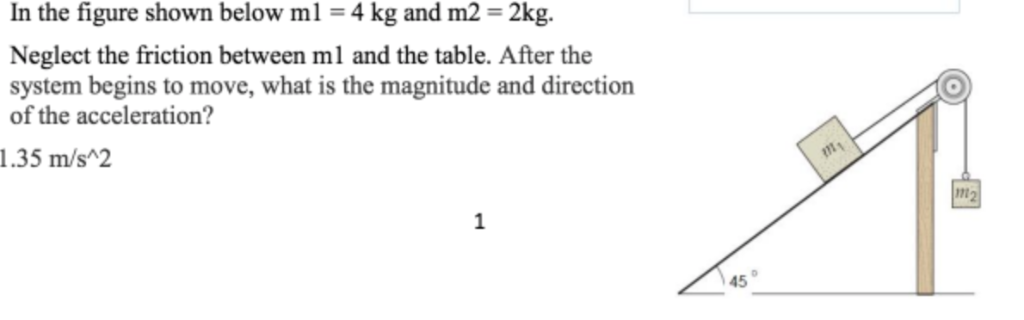In the figure shown below ml-4 kg and m2- 2kg. Neglect the friction between ml and the table, After the system begins to move, what is the magnitude and direction of the acceleration? 0 1.35 m/s2 1n 45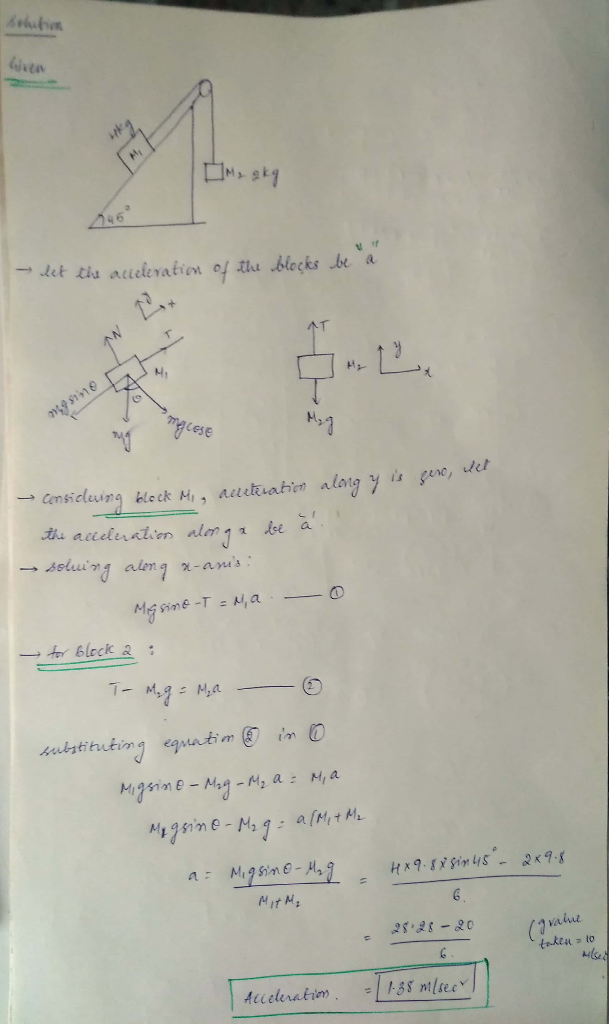#### Earn Coin

Coins can be redeemed for fabulous gifts.

Similar Homework Help Questions
• ### In the figure below, m1 = 10.0 kg and m2 = 4.5 kg. The coefficient of...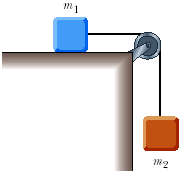In the figure below, m1 = 10.0 kg and m2 = 4.5 kg. The coefficient of static friction between m1 and the horizontal surface is 0.60 while the coefficient of kinetic friction is 0.30. (a) If the system is released from rest, what will its acceleration be? m/s2 (b) If the system is set in motion with m2 moving downward, what will be the acceleration of the system? m/s2

• ### m2 mi h - 2 kg, m2 Problem 1 refers to the figure below. In the...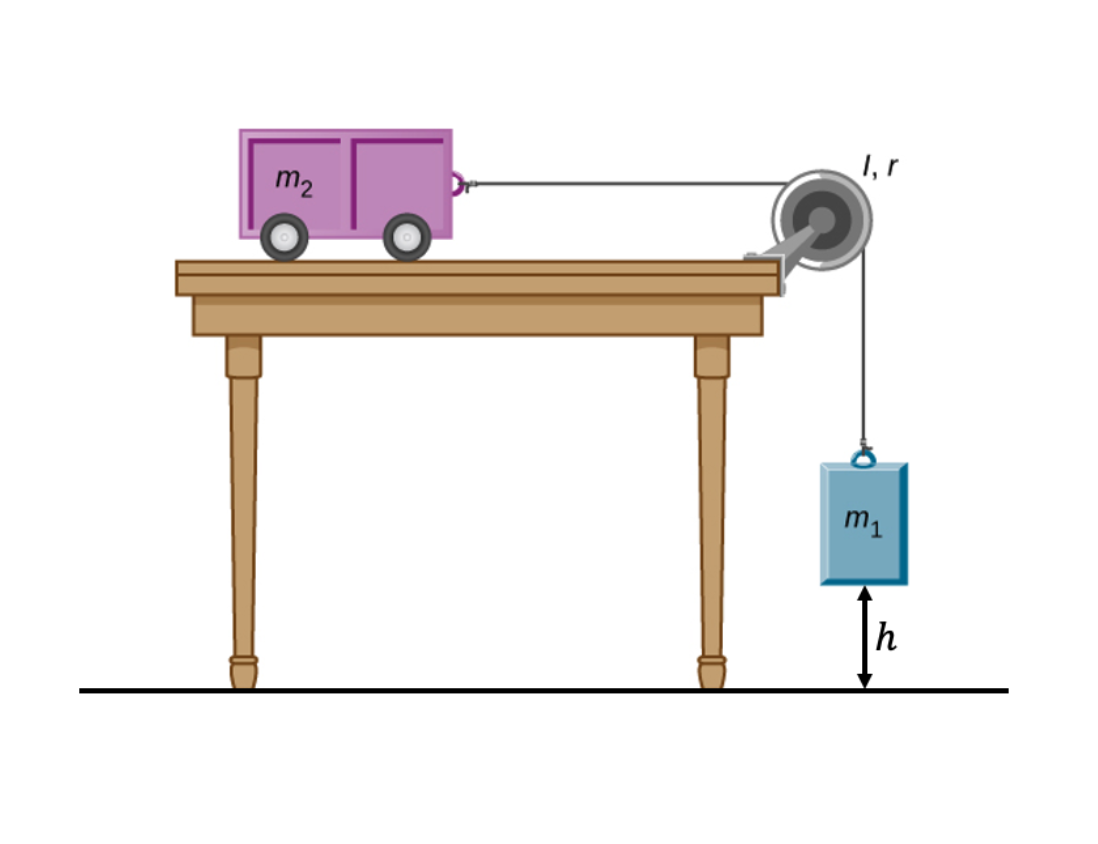m2 mi h - 2 kg, m2 Problem 1 refers to the figure below. In the figure below m2 is attached to a rope that goes over pulley and then the rope is attached to mi. mi 4 kg, and the pulley has a moment of inertia I = 0.4 kg · m2 and a radius r = 0.2 kg. mi, m2, and the pulley are initially at rest. There is no friction between my and the table and my...

• ### An aluminum block of mass mı = 3.85 kg and a copper block of mass m2...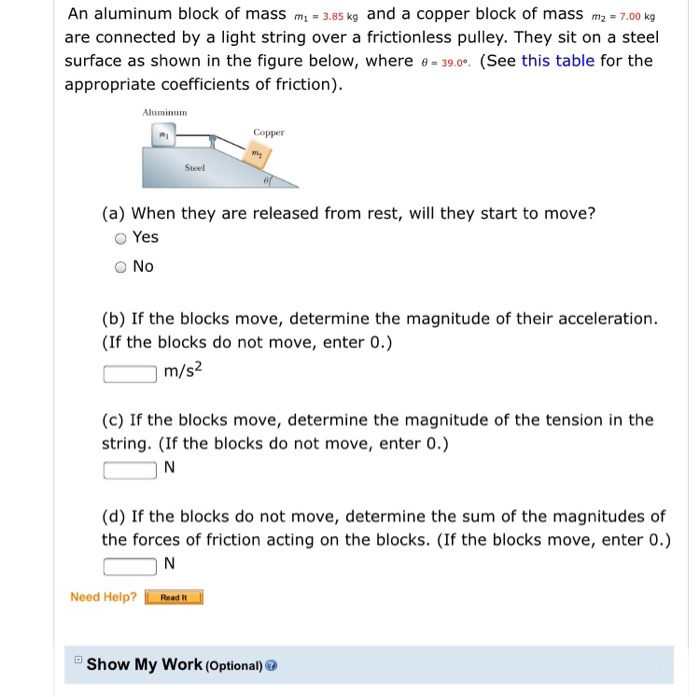An aluminum block of mass mı = 3.85 kg and a copper block of mass m2 = 7.00 kg are connected by a light string over a frictionless pulley. They sit on a steel surface as shown in the figure below, where e = 39.09. (See this table for the appropriate coefficients of friction). Aluminum Copper Swel (a) When they are released from rest, will they start to move? Yes No (b) If the blocks move, determine the magnitude of...

• ### 4 From the figure below, ml - 2 kg, m2 -3 kg, m3-10 kg, p tem....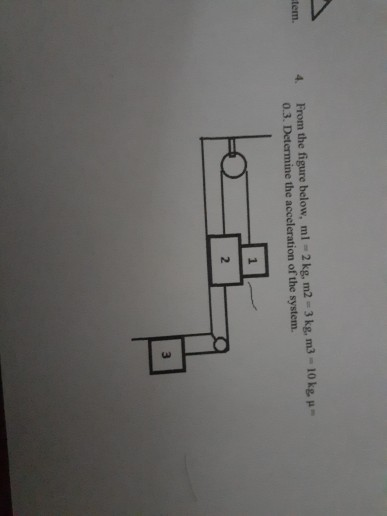4 From the figure below, ml - 2 kg, m2 -3 kg, m3-10 kg, p tem. 0.3. Determine the acceleration of the system. 2

• ### The cart shown below moves across the table top as the block falls. what is the...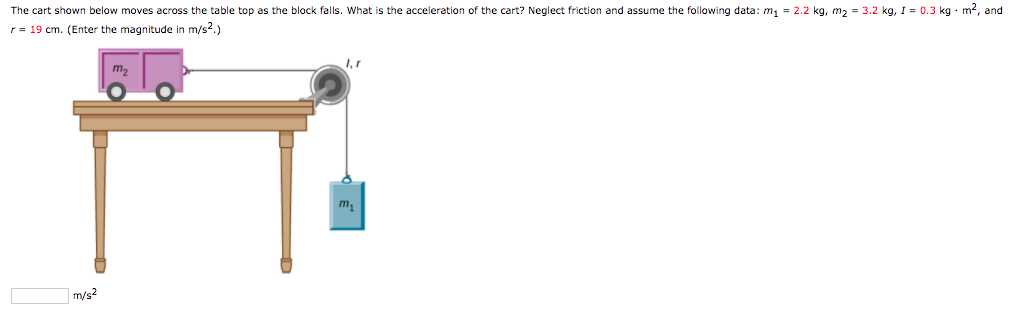The cart shown below moves across the table top as the block falls. what is the acceleration of the cart? Neglect friction and assume the following data: m1-2.2 kg, m2 = 3.2 kg, 1-0.3 kg·m2, and r= 19 cm. (Enter the magnitude in m/s2.)

• ### Two masses M1=2kg and M2 are attached by a massless cord over a solid pulley wheel...

Two masses M1=2kg and M2 are attached by a massless cord over a solid pulley wheel of mass M=4kg, and radius R=5cm. Static Friction between the cord and the pulley makes the pulley rotate counter-clockwise when the system is released from rest, M1 accelerates with a magnitude of 3.92 m/s2. a) Draw and label the forces acting on the two blocks, and the pulley. (6 points) b) Find the tension in the cord between the pulley and M1 (6 points)...

• ### 3. 35 points Two blocks (m1 = 2kg, m2 = 4kg) are connected over a pulley...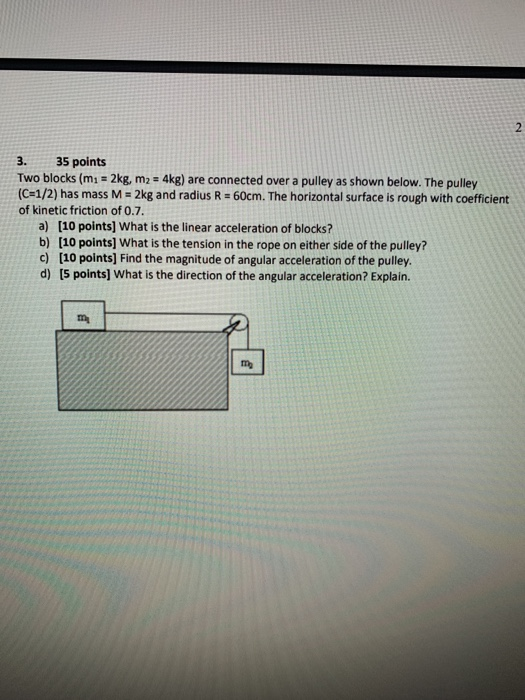3. 35 points Two blocks (m1 = 2kg, m2 = 4kg) are connected over a pulley as shown below. The pulley (C=1/2) has mass M = 2kg and radius R = 60cm. The horizontal surface is rough with coefficient of kinetic friction of 0.7. a) [10 points] What is the linear acceleration of blocks? b) (10 points) What is the tension in the rope on either side of the pulley? c) (10 points) Find the magnitude of angular acceleration of...

• ### A block of mass m2 = 38 kg on a horizontal surface is connected to a...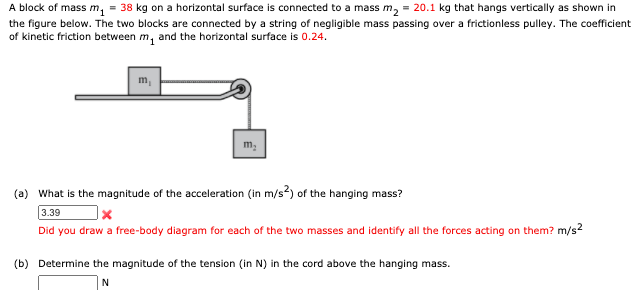A block of mass m2 = 38 kg on a horizontal surface is connected to a mass m2 = 20.1 kg that hangs vertically as shown in the figure below. The two blocks are connected by a string of negligible mass passing over a frictionless pulley. The coefficient of kinetic friction between m, and the horizontal surface is 0.24. m (a) What is the magnitude of the acceleration (in m/s2) of the hanging mass? 3.39 Did you draw a free-body...

• ### Forces/kinetic friction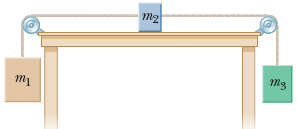Three blocks are connected on the table as shown below. The coefficient of kinetic friction between the block of mass m2 and the table is 0.420. The objects havemasses of m1 = 4.50 kg, m2 = 1.50 kg, and m3 = 2.50 kg, and the pulleys are frictionless.(b) Determine the acceleration of each object, including its direction.am1:magnitudem/s2direction---Select--- up downam2:magnitudem/s2direction---Select--- left rightam3:magnitudem/s2direction---Select--- down up(c) Determine the tensions in the two cords.left cordNright cordN(d) If the tabletop were smooth, would the tensions...

• ### 2. A cube and a wedge with masses m1-5.00 kg and m2 10.0 kg are arranged...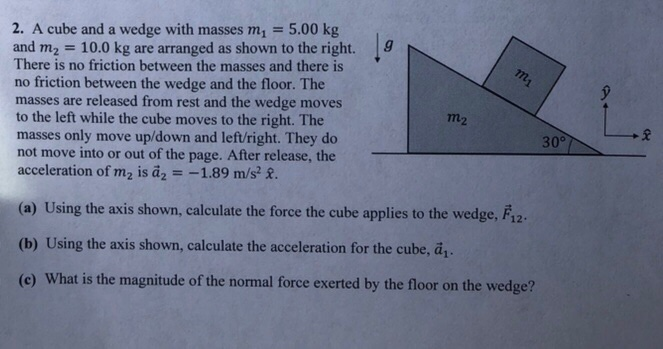2. A cube and a wedge with masses m1-5.00 kg and m2 10.0 kg are arranged as shown to the right. 9 There is no friction between the masses and there is no friction between the wedge and the floor. The masses are released from rest and the wedge moves to the left while the cube moves to the right. The masses only move up/down and left/right. They do not move into or out of the page. After release, the...